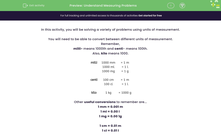# Understand Measuring Problems

In this worksheet, students will be asked to solve measurement problems using mixed units and will then convert between units.Key stage:  KS 2

Curriculum topic:   Measurement

Curriculum subtopic:   Solve Conversion Problems

Difficulty level:#### Worksheet Overview

In this activity, you will be solving a variety of problems using units of measurement.

You will need to be able to convert between different units of measurement.

Remember,

milli- means 1000th and centi- means 100th.

Also, kilo means 1000.

 milli 1000 mm = 1 m 1000 ml = 1 l 1000 mg = 1 g centi 100 cm = 1 m 100 cl = 1 l kilo 1 kg = 1000 g

Other useful conversions to remember are...

1 mm = 0.001 m

1 ml = 0.00 l

1 mg = 0.00 1g

1 cm = 0.01 m

1 cl = 0.01 l

1 g = 0.001 kg

Reminder:

When solving a problem that uses more than one unit of measurement, always convert them so you are just working in one unit.

It's usually easier to work in the smaller unit and then convert back at the end if the question asks for the answer in the larger unit of measurement.

Example:

Zach pours 5 litres of water into his paddling pool. He then removes 600 ml. Finally, he adds another 50 cl.

How much water is now in the paddling pool?

Work in millilitres

5000 ml - 600 ml + 500 ml = 4900 ml

Convert back to litres by dividing by 1000.

4.9 l is in the paddling pool.

Your turn!

### What is EdPlace?

We're your National Curriculum aligned online education content provider helping each child succeed in English, maths and science from year 1 to GCSE. With an EdPlace account you’ll be able to track and measure progress, helping each child achieve their best. We build confidence and attainment by personalising each child’s learning at a level that suits them.

Get started